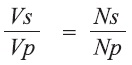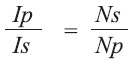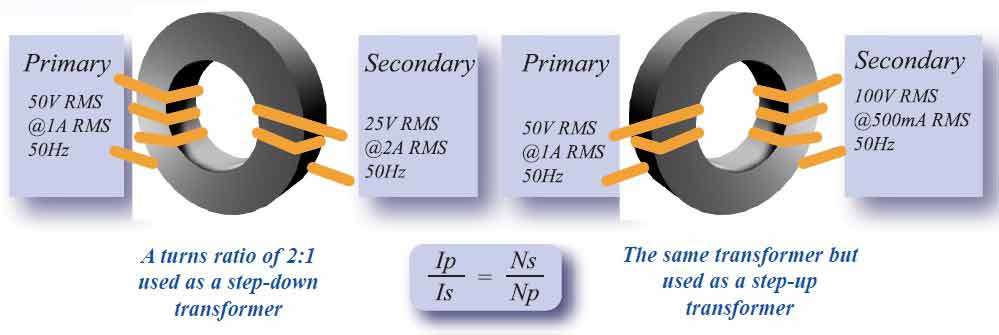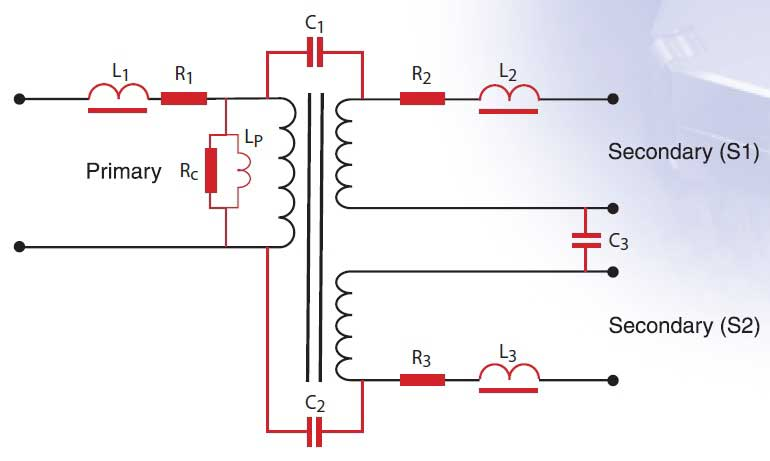This is a machine translation, and may not be perfect in all cases.

You can turn this off at any time, and return to the original English by selecting “English” at the top of the drop down menu.

# Turns Ratio - Types of Test Available

An explanation of Turns Ratio and several methods to obtain best measurements

## 1, Introduction to Turns Ratio

Transformers are used in a wide array of electrical or electronic applications, providing functions that range from isolation and stepping up or stepping down voltage and current to noise rejection, signal measurement, regulation and a host of functions particular to specific applications.

In order to test that a transformer will meet its design specification, a number of functions should be tested and one of the most commonly used tests is turns ratio.

This technical note will review briefly the basic theory of turns ratio and then introduce some additional issues that should be considered when testing this critical transformer characteristic.

## 2, Basic Theory

The turns ratio of a transformer is defined as the number of turns on its secondary divided by the number of turns on its primary.

The voltage ratio of an ideal transformer is directly related to the turns ratio:The current ratio of an ideal transformer is inversely related to the turns ratio:Where Vs = secondary voltage, Is = secondary current, Vp = primary voltage, Ip = primary current, Ns = number of turns in the secondary winding and Np = number of turns in the primary winding.

The turns ratio of a transformer therefore defines the transformer as step up or step-down.
A step-up transformer is one whose secondary voltage is greater than its primary voltage and a transformer that steps up voltage will step-down current.
A step-down transformer is one whose secondary voltage is lower than its primary voltage and a transformer that steps down voltage will step-up current.

### Voltage and Current Turns Ratio Definitions## 3, Factors Affecting Turns Ratio Measurements

With a theoretical, "ideal" transformer, the ratio of the physical turns on any winding could be established simply by measuring the RMS output voltage on one winding, while applying a known RMS input voltage of an appropriate frequency to another winding.

Under these conditions, the ratio of the input to output voltages would be equal to the physical turns ratio of these windings.
Unfortunately, however, "real" transformers include a number of electrical properties that result in a voltage or current ratio that may be not equal to the physical turns ratio.
The following schematic diagram illustrates the electrical properties of a real transformer, with the ideal transformer component shown in the centre, plus the electrical components that represent various additional properties of the transformer.• L1, L2 and L3 represent the primary and secondary leakage inductance caused by incomplete magnetic coupling between the windings.
• R1, R2 and R3 represent the resistance (or copper loss) of the primary and secondary windings.
• C1, C2, and C3 represent the interwinding capacitance.
• Lp represents the magnetizing inductance core loss.
• Rp represents the core loss of which three areas contribute, eddy current loss (increases with frequency), hysteresis loss (increases with flux density) and residual loss (partially due to resonance).

## 4, Types of Turns Ratio Tests

When considering the range of elements shown in the transformer schematic and considering also the varying requirements of different transformer applications, it can be seen that no single measurement technique will fully satisfy all turns ratio questions.
For this reason, Voltech AT series transformer testers offer five different turns ratio measurement techniques, which can be selected individually to meet specific needs.
The basic specifications and Voltage/ frequency range of measurement can be seen on our AT5600 Specification Page

TR (turns ratio)
This test energizes any chosen winding at a specified voltage and measures the induced voltage on any other winding.
The results are then presented as a ratio (e.g. 2:1, 5:1, etc.) Voltech AT testers do this by dividing one voltage by the other while compensating for winding resistance.
Phase is also measured: 'in-phase' (positive polarity) and 'anti-phase' (negative polarity).
See the AT5600 User Manual page

TRL (turns ratio by inductance)
This test separately energizes two selected windings and measures the inductance value of each winding.
The results are then presented as a ratio of turns (e.g. 2:1, 5:1, etc.) calculated from the squared root of the inductance values.
Phase is also: 'in- phase' (positive polarity) and 'anti-phase' (negative polarity).
See the AT5600 User Manual page

LVOC (low voltage open circuit)
This test applies a voltage to the primary winding, reads the voltage induced in the secondary winding and presents the results as a secondary voltage (e.g. 2.545 V).
Phase is also measured: 'in- phase' (positive polarity) and 'anti-phase' (negative polarity).
See the AT5600 User Manual page

VOC (voltage open circuit - AT5600 + AT3600 only)
This test uses the same principal as LVOC but by using a high-power generator, capable of energizing a winding at voltages up to 270V.
The test is suitable for testing low-frequency power transformers.
Phase is also measured: 'in- phase' (positive polarity) and 'anti-phase' (negative polarity).
See the AT5600 User Manual page

VOCX (voltage open circuit with external source - AT5600 + AT3600 only)
This test, which is used in conjunction with the Voltech AC Interface Fixture.
This will control an external AC source or step-up transformer for testing higher power and higher voltage transformers up to 600V and 10A.
Phase is also measured: 'in-phase' (positive polarity) and 'anti-phase' (negative polarity).
See the AT5600 User Manual page

## 5, Choosing the Correct Turns Ratio Test

In order to determine which type of turns ratio test is most appropriate for a particular transformer, a number of issues should be considered.
The table below shows each test with a description, the related specifications and a summary of the benefit provided by that test.

#### Use or Benefit

TR

Ratio of input to output volts

Measurement range: 1:30 to 30:1 Voltage range: 1 mV - 5V Frequency range: 20 Hz - 3MHz Accuracy: 0.1%

Shows the true electrical ratio as expected in operation when energizing a primary winding.

The ratio measured with this test therefore includes the losses normally found in the transformer, which will result in a ratio greater than that of the physical turns but reflects the real voltage ratio expected by the designer.

TRL

Ratio of turns calculated from inductance

Measurement range: 1:30 to 30:1 Voltage range: 1 mV - 5V Frequency range: 20 Hz - 3MHz Accuracy: 0.1%

Reduces the effect on measured turns ratio of transformer losses, giving a closer approximation to the physical turns ratio.

This is of particular benefit where actual turns are of interest but the transformer has a large proportion of leakage inductance which may have a significant effect on the voltage ratio.

LVOC

Output volts measured with low-voltage input

Measurement range: 100μV to 650 V (100μV to 5V ATi) Voltage range: 1 mV - 5V Frequency range: 20 Hz - 3MHz Accuracy: 0.1%

Similar to TR but presents the actual output voltage rather than the voltage ratio.

This simplifies test limit entry when the transformer specification has been derived from voltmeter measurements.

VOC

Output volts measured with external high-voltage input

Measurement range: 100μV to 650 V Voltage range: 5V - 600V Frequency range: 20 Hz - 1 MHz Accuracy: 0.1%

Provides the ability to test power transformers that are above the capacity of VOC testing.

By controlling an external power source with the Voltech AC Interface Fixture, the VOCX test provides fully automatic testing of high-power transformers at their specified working voltage.

VOCX

Output volts measured with external high-voltage input

Measurement range: 100μV to 650V
Voltage range: 5V - 600V
Frequency range: 20Hz - 1MHz
Accuracy: 0.1%

Provides the ability to test power transformers that are above the capacity of VOC testing.

By controlling an external power source with the Voltech AC Interface Fixture, the VOCX test provides fully automatic testing of high-power transformers at their specified working voltage

## 6, Conclusion On Testing for Turns Ratio

While turns ratio may be a well known and very fundamental function in a transformer, it can be seen that testing this function effectively requires the consideration of many issues.

By providing a flexible range of turns ratio test options, the Voltech AT5600 provides designers and manufacturers alike with the opportunity to select the most appropriate tests for any transformer design and therefore optimise the quality and efficiency of their test process.

Should you have questions on any of the other test functions available for the Voltech AT series transformer testers, please do not hesitate to contact us.